Hydrogen Emission Spectrum Chemistry Tutorial

Key Concepts

• An electron in the ground state can absorb energy and enter a higher energy level (excited state).

For hydrogen, an electron in the ground state occupies the first energy level (n=1)

For hydrogen, an electron in the excited state occupies an energy level greater than n=1 (ie, n=2, n=3 etc)

• An excited electron will fall down to a lower energy level, emitting a photon of particular energy (and hence of a particular wavelength(1)) in the process.

The greater the energy of the photon emitted, the shorter its wavelength is.

• Observing and recording the wavelengths of these emitted photons results in an emission spectrum consisting of discrete lines.
• The lines in the hydrogen emission spectrum are grouped together in a number of different named series:

⚛ Lyman series : a group of lines in the ultraviolet region of the electromagnetic spectrum.

⚛ Balmer series : a group of lines around the visible region of the electromagnetic spectrum.

⚛ Paschen series : a group of lines in the infrared region of the electromagnetic spectrum.

⚛ Brackett series, Pfund series and Humphreys series also occur in the infrared region of the electromagnetic spectrum.

• Each of these series corresponds to excited electrons falling down to a particular energy level:(2)

⚛ Lyman series : excited electrons fall back to the n=1 energy level

⚛ Balmer series : excited electrons fall back to the n=2 energy level

⚛ Paschen series : excited electrons fall back to the n=3 energy level

⚛ Brackett series : excited electrons fall back to the n=4 energy level

⚛ Pfund series : excited electrons fall back to the n=5 energy level

⚛ Humphreys series : excited electrons fall back to the n=6 energy level

No ads = no money for us = no free stuff for you!

Ground State and Excited State

A hydrogen atom has just one proton (positive charge) in its nucleus and one electron (negative charge) "orbiting" the nucleus.
If we could look at the structure of a hydrogen atom in the air at 25°C and 100 kPa, then we would see that this electron occupies the first energy level, or K electron shell. This is known as the ground state for this electron.
The first energy level (K shell) is represented by the principal quantum number (n) 1, that is, n=1
The diagram below can be used to describe the hydrogen atom when the electron (e) is in its ground state (n=1):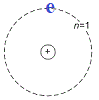On earth we don't find hydrogen atoms existing on their own, but we can find hydrogen gas which is a diatomic molecule made up of 2 hydrogen atoms sharing their electrons to form a covalent bond, that is, hydrogen gas has the molecular formula H2(g).

If we place a sample of hydrogen gas (H2(g)) in a gas discharge tube and pass an electric current through it, then the energy supplied will break the covalent bond so that we have individual hydrogen atoms existing in the gas dishcarge tube.
The hydrogen molecule (H2(g)) is said to dissociate into hydrogen atoms and each hydrogen atom has 1 electron ():

Dissociation: H2(g) → 2H(g)

But the electron in each hydrogen atom can also absorb energy from the electrical energy supplied in the gas discharge tube! When an electron absorbs energy it will move faster which means it will no longer be stable in the n=1 energy level (K shell). This faster electron moves further away from the nucleus, it is said to jump to a higher energy level.
This electron is said to be "excited", it exists in a higher energy level than it does in the non-excited or ground state.

Only energy levels (shells) with particular energy values are available to the excited electron.
Higher energy levels are represented by higher principal quantum numbers, n=2, n=3, n=4 etc
The diagram below shows some of the energy levels available to the excited electron in a hydrogen atom: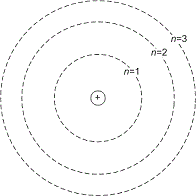In its ground state, hydrogen's electron occupies the n=1 energy level.
But if the electron is excited enough it can absorb enough energy it could jump to the n=2 level. This is shown on the diagram above by an arrow starting at the n=1 ground state and pointing to the n=2 energy level for the excited state: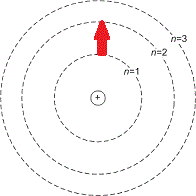If it absorbs even more energy it could jump to the n=3 level, as shown in the diagram below: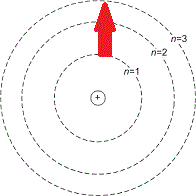But there is an upper limit to how much energy this electron can absorb because it can absorb so much energy that it is moving so fast it will move so far away from the nucleus that it escapes entirely!
The hydrogen atom then loses the electron and becomes ionised. This ionisation is represented as:

Ionisation: H(g) → H+(g) + e-

This "upper limit" to the available energy levels is given the principal quantum number of infinity, n=∞

To represent an electron transition from one energy level to another we don't usually draw all those circles, we usually represent each energy level (shell) with a single line as shown in the diagram below:

Principal Quantum Number Energy Level Relative Energy
n=4
high

n=3

n=2

n=1
low

An electron in the n=4 energy level has more energy than an electron in the n=3 energy level.
An electron in the n=3 energy level has more energy than an electron in the n=2 energy level, etc.

Note that as the energy levels increase in energy (and increase in principal quantum number) they get closer together.
The difference in energy between n=1 and n=2 is greater than the difference in energy between n=2 and n=3.

Do you know this?

Play the game now!

Emission Spectrum for Hydrogen

We saw above that electrons in the hydrogen atom become excited in a gas discharge tube, they absorb a quanta of energy and jump to a higher energy level.
But these excited electrons do not stay excited! They lose energy by emitting a photon of light and drop back to a lower energy level (with a lower principal quantum number).

Imagine an excited electron that exists in the n=4 energy level as shown in the diagram below:

Principal Quantum Number Energy Level Relative Energy
n=4 e
high

n=3

n=2

n=1
low

This excited electron could lose a bit of energy and fall back down to the n=3 energy level:

Principal Quantum Number Energy Level Relative Energy
n=4
high

n=3

n=2

n=1
low

The excited electron loses energy by emitting a photon of light with energy equal to the difference in energy between the n=4 and n=3 energy levels.
This photon will have a particular wavelength (or frequency) determined by its energy.

Or it could emit even more energy and fall back to the n=2 level, or emit even more energy still and fall back to the ground state the n=1 energy level:

Principal Quantum Number Energy Level Transition Energy Level Transition Energy Level Transition
n=4

n=4 to n=3 |
|
|
|
n=4 to n=2 |
|
|
|
|
|
|
|
n=4 to n=1
n=3

n=2

n=1

A photon of light emitted during the n=4 to n=3 transition will have less energy than a photon of light emitted during the n=4 to n=2 transition.
The more energy the photon has, the greater its frequency and the shorter its wavelength is.
High energy photon ≡ shorter wavelength (high energy photon ≡ higher frequency)
Low energy photon ≡ longer wavelength (low energy photon ≡ lower frequency)

Furthermore, it is possible for an excited electron in the n=3 energy level to lose a quanta of energy and fall back to the n=2 energy level, or lose even more energy and fall back to the n=1, ground state, energy level!
There are lots of possible transitions!
And each time an excited electron loses energy it emits a photon of light with a particular amount of energy, and a particular wavelength and frequency.
Using intruments like a spectroscope, we can see a series of lines on a dark (black) background which is referred to as an emission spectrum.
A section of the emission spectrum for hydrogen is shown below: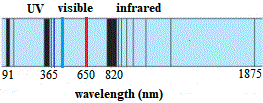Each line on the emission spectrum for hydrogen corresponds to the wavelength (or frequency) of an emitted photon of light with the energy equivalent to the loss of energy when the excited electron dropped down to one of the lower, allowed, energy levels.
Note that some lines in the emission spectrum correspond to wavelengths of light in the ultraviolet (UV) region of the electromagnetic spectrum, some occur in the visible region of the electromagnetic spectrum, others occur in the infrared region of the electromagnetic spectrum.
Scan the emission spectrum from right to left (from 1875 nm to 820 nm).
Can you see how the distance between the lines gets smaller until the distance between them is so small that all the lines "bunch up" and created what looks like one broad line?
Then a new line appears in the visible region (656 nm), but once again the distance between the lines start to decrease again until we reach 365 nm and all the lines are so close together that we can't differentiate between them and they look like one broad band.
It looks like the same thing is happening in the UV region, one line then a broad band of lines too close together to be differentiated.
Each of these groups of lines is referred to as a series and has been named.
The group of lines shown above in the infrared is refered to as the Paschen series, the group in the visible area is the Balmer series, and the group in the UV area are known as the Lyman series.

Do you understand this?

Take the test now!

Lyman Series (ultraviolet)

The Lyman Series is a group of lines in the ultraviolet portion of the emission spectrum of the hydrogen atom, named after their discoverer Theodore Lyman who found these lines between 1906 and 1914.

These lines occur when the excited electron falls back from a higher energy level (n ≥ 2) to the first energy level (n=1).

The wavelengths of light associated with the electron transitions in the Lyman series are given below:

 Transition wavelength (nm) n=2 to n=1 n=3 to n=1 n=4 to n=1 n=5 to n=1 n=6 to n=1 n=7 to n=1 n=8 to n=1 n=9 to n=1 n=10 to n=1 n=11 to n=1 n=∞ to n=1 121.6 102.6 97.3 95.0 93.8 93.1 92.6 92.3 92.1 91.9 91.8

Note that the n=∞ to n=1 transition represents the limit of the Lyman Series, because the electron would have so much energy at this point that it would escape from the attractive pull of the nucleus and the hydrogen atom would become ionised, that is, the atom would have lost the electron.

An electron in the n=10 energy level has more energy than an electron in the n=9 energy level.
The transition from n=10 to n=1 involves emitting a photon of much greater energy than the n=9 to n=1 transition for example, so the wavelength of the emitted photon of light for the n=10 to n=1 is shorter than the wavelength of the photon emitted for the n=9 to n=1 transition.

Let's label some of the lines in the Lyman series of the hydrogen emission spectrum with the corresponding electron transitions:

The higher the energy of the excited electron, the higher the energy level it occupies.
The higher the energy of the excited electron (the greater the value of n), the greater the energy that it loses when it falls back to the ground state (n=1).
Since the electron loses energy by emitting a photon, the greater the energy the electron loses, the greater the energy of the emitted photon and hence the shorter its wavelength will be.

Balmer Series (visible)

The Balmer series of lines in the hydrogen emission spectrum, named after Johann Balmer, is a set of 4 lines that occur in the visible region of the electromagnetic spectrum as shown below: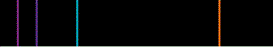and a number of additional lines in the ultraviolet region.

These lines occur when an excited electron (n≥3) falls back down to the n=2 energy level.(3)

The wavelengths of light associated with some of the electron transitions in the Balmer series are given below:

 Transition wavelength (nm) region n=3 to n=2 n=4 to n=2 n=5 to n=2 n=6 to n=2 n=7 to n=2 n=8 to n=2 n=9 to n=2 n=10 to n=2 n=∞ to n=2 656 486 434 410 397 389 384 380 364.6 visible ultraviolet

Note that the n=∞ to n=2 transition represents the limit of the Balmer Series, because the electron would have so much energy at this point that it would escape from the attractive pull of the nucleus and the hydrogen atom would become ionised, that is, the atom would have lost the electron.(4)

An electron in the n=10 energy level has more energy than an electron in the n=9 energy level.
The transition from n=10 to n=2 involves emitting a photon of much greater energy than the n=9 to n=2 transition for example, so the wavelength of the emitted photon of light for the n=10 to n=2 is shorter than the wavelength of the photon emitted for the n=9 to n=2 transition.

Let's label some of the lines in the Balmer series of the hydrogen emission spectrum with the corresponding electron transitions:

The higher the energy of the excited electron, the higher the energy level it occupies.
The higher the energy of the excited electron (the greater the value of n), the greater the energy that it loses when it falls back to the n=2 energy level.
Since the electron loses energy by emitting a photon, the greater the energy the electron loses, the greater the energy of the emitted photon and hence the shorter its wavelength will be.

Paschen Series (infrared)

The Paschen series of lines in the hydrogen emission spectrum occurs in the infrared region of the electromagnetic spectrum and is named after Friedrich Paschen who was the first to observe these lines in 1908.

These lines occur when an excited electron falls from a higher energy level (n ≥ 4) back down to the n=3 energy level.

The wavelengths of light associated with some of the electron transitions in the Paschen series are given below:

 Transition wavelength (nm) n=4 to n=3 n=5 to n=3 n=6 to n=3 n=7 to n=3 n=8 to n=3 n=9 to n=3 n=10 to n=3 n=∞ to n=3 1875 1282 1094 1005 955 923 902 820

Note that the n=∞ to n=3 transition represents the limit of the Paschen Series, because the electron would have so much energy at this point that it would escape from the attractive pull of the nucleus and the hydrogen atom would become ionised, that is, the atom would have lost the electron.

An electron in the n=10 energy level has more energy than an electron in the n=9 energy level.
The transition from n=10 to n=3 involves emitting a photon of much greater energy than the n=9 to n=3 transition for example, so the wavelength of the emitted photon of light for the n=10 to n=3 is shorter than the wavelength of the photon emitted for the n=9 to n=3 transition.

Let's label some of the lines in the Paschen series of the hydrogen emission spectrum with the corresponding electron transitions:

The higher the energy of the excited electron, the higher the energy level it occupies.
The higher the energy of the excited electron (the greater the value of n), the greater the energy that it loses when it falls back to the n=3 energy level.
Since the electron loses energy by emitting a photon, the greater the energy the electron loses, the greater the energy of the emitted photon and hence the shorter its wavelength will be.

Can you apply this?

Join AUS-e-TUTE!

Take the exam now!

Other Series (even longer wavelengths!)

Based on what we saw above we would predict that there will be more series of lines at even longer wavelengths in the hydrogen emission spectrum, corresponding to excited electrons falling from higher energy levels to the n=4 energy level, and from higher energy levels to the n=5 energy level etc.
And indeed, this is true.

Brackett Series

The Brackett series of lines, first observed by Frederick Sumner Brackett in 1922, results when an excited electron falls from a higher energy level (n ≥ 5) to the n=4 energy level.
The wavelengths of some of the emitted photons during these electron transitions are shown below:

 Transition wavelength (nm) n=5 to n=4 n=6 to n=4 n=7 to n=4 n=8 to n=4 n=9 to n=4 n=10 to n=4 n=∞ to n=4 4051 2625 2166 1944 1817 1736 1458

Pfund Series

The Pfund series of lines, first observed by August Herman Pfund in 1924, results when an excited electron falls from a higher energy level (n ≥ 6) to the n=5 energy level.
The wavelengths of some of the emitted photons during these electron transitions are shown below:

 Transition wavelength (nm) n=6 to n=5 n=7 to n=5 n=8 to n=5 n=9 to n=5 n=10 to n=5 n=∞ to n=5 7460 4654 3741 3297 3039 2279

Humphreys Series

The Humphreys series of lines, first observed by Curtis J. Humphreys in 1953, results when an excited electron falls from a higher energy level (n ≥ 7) to the n=6 energy level.
The wavelengths of some of the emitted photons during these electron transitions are shown below:

 Transition wavelength (nm) n=7 to n=6 n=8 to n=6 n=9 to n=6 n=10 to n=6 n=∞ to n=6 12370 7503 5908 5129 3282

Footnotes:

(1) Energy of the photon emitted (E) equals Planck's constant (h) multiplied by its frequency (ν): E = hν
The frequency of this photon of light (ν) multiplied by its wavelength (λ) equals its speed (c): c = λν
So, rearranging this equation gives ν = c/λ
Substituting this expression for ν in the first equation for energy: E = (hc)/λ
hc represents two constants multiplied together.
Therefore energy is inversely proportional to wavelength: E ∝ 1/λ
If energy (E) increases then wavelength (λ) decreases.
If energy (E) decreases then wavelength (λ) increases.

(2) Do you need to remember which series is which? We have some suggestions.
Let's just think about the first three: Lyman, Balmer, Paschen, series
L looks a lot like 1, so the Lyman series has excited electrons falling down to the n=1 ground state energy level. (LUV Lyman, so this is in the UV region)
B is the second letter of the alphabet, so the Balmer series has excited electrons falling down to the second energy level, n=2 (Balmy days when the sun is out, so this is in the visible region)
P is remaining so the Paschen series is the third in sequence, excited electrons are falling down to the third energy level, n=3, and it occurs in the infrared region (ir).

(3) Balmer discovered a relationship between the wavelength (λ) of the lines in this series and the principal quantum numbers (n) that produce them:
(1/λ) = RH(1/22 − 1/n2)
RH = Rydberg constant = 1.09677576 × 107 m-1 (for hydrogen)
We can use this relationship to calculate the wavelength of emitted photons and then construct an emission spectrum based on these calculations.
This relationship can be applied to the other series by changing the first term in the round bracket to the principal quantum number of the energy level the excited electrons are falling down to:
Lyman series: (1/λ) = RH(1/12 − 1/n2)
Balmer series: (1/λ) = RH(1/22 − 1/n2)
Paschen series: (1/λ) = RH(1/32 − 1/n2)
Brackett series: (1/λ) = RH(1/42 − 1/n2)
Pfund series: (1/λ) = RH(1/52 − 1/n2)
Humphreys series: (1/λ) = RH(1/62 − 1/n2)

(4) Ionisation of hydrogen gas involves removing an electron in the ground state, that is, the electron transition involved is from n=1 to n=∞
Using the relationship above we can calculate the wavelength of light required:
(1/λ) = RH(1/12 − 1/∞2)
1/∞2 → 0 and 1/12 = 1
So the expression becomes: (1/λ) = RH = 1.09677576 × 107 m-1
therefore: λ = 9.1176 × 10-8 m
which we can use to determine the energy required to pull off the electron (to ionise the hydrogen atom):
E = (hc)/λ
h = 6.626 × 10-34
c = 3 × 108
E = (6.626 × 10-34 × 3 × 108)/9.1176 × 10-8 = 2.18 × 10-18 J (per hydrogen atom)
Ionisation energy (energy required to remove an electron from a gaseous atom) is usually given in kJ mol-1, so for hydrogen:
E = 2.18 × 10-18 J atom-1 = 2.18 × 10-21 kJ atom-1
E = (2.18 × 10-21 kJ atom-1) × (6.02 × 1023 atoms mol-1) = 1312 kJ mol-1 (1310 kJ mol-1 to 3 significant figures)
This is in good agreement with published values for the measurement of ionisation energy for hydrogen, 1312 kJ mol-1